# Power Query – Percent of total or categoryIn this post, I want to cover how to calculate the percent of a total in Power Query.  We’ll also take this one step further, to consider how to calculate the percent of a category.

In standard Excel, these calculations are simple, as we are so used to the formulas to achieve this.  But for many, Power Query is still a newer tool, and it doesn’t operate in quite the same way.

I recommend you download the example file for this post.  Then you’ll be able to work along with examples and see the solution in action, plus the file will be useful for future reference.

Contents

The data in our example looks like this; it is a list of cities where a company has offices.  Each office has a region category and a headcount value.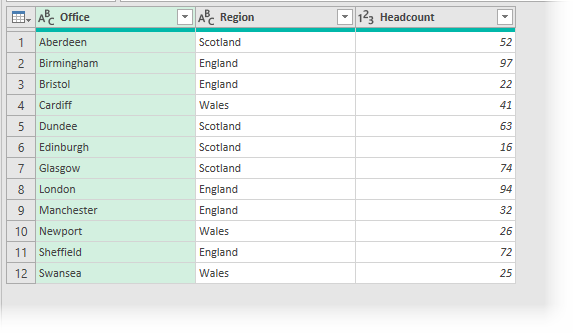### Percent of the total in Power Query

To calculate the % of the total is reasonably straight forward.  While the SUM function does not exist in Power Query, the List.Sum function does.

1. Within Power Query click Add Column -> Custom Column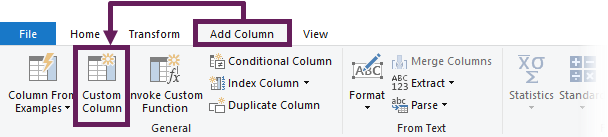2. In the Custom Column dialog box enter the following formula:
`=[Headcount] / List.Sum(#"Changed Type"[Headcount])`
3. Change the formula to fit your scenario:
• [Headcount] is the name of the column for which you want to calculate the %
• #”Changed Type” is the name of the step to be used as the source for the formula. Typically, this is the name of the previous step in the Advanced Editor window.
4. Give the custom column a useful name, such as % of total, then click OK.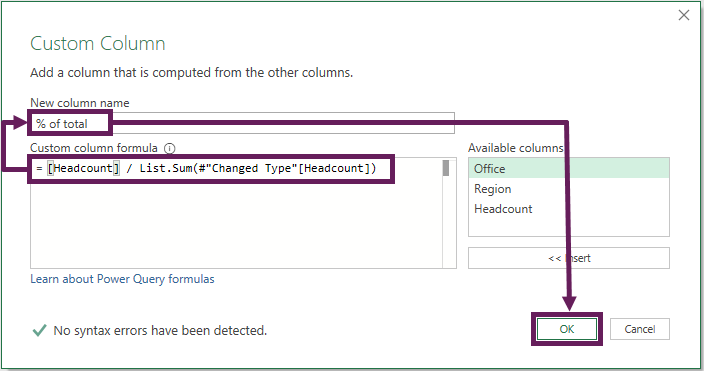5. The % of total column will now be included in the preview window.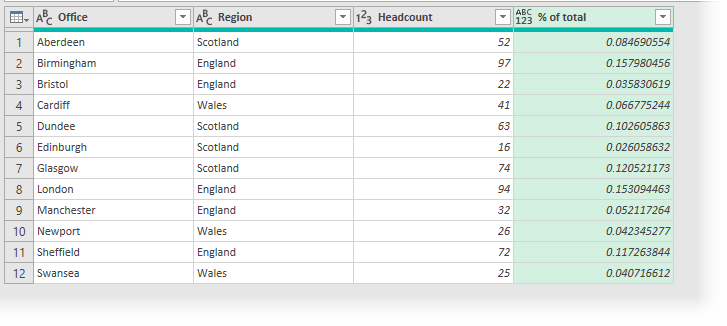6. It doesn’t look like a percentage yet, so change the data type by clicking on the ABC123 button and selecting Percentage from the menu.That’s it, here is the final table: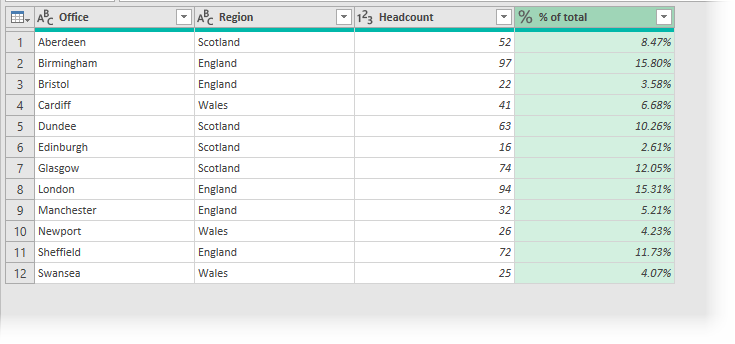That wasn’t too bad, was it, eh?  The solution was very similar to how we would do it in Excel.

### Percent of category in Power Query

To calculate the % of a category, it’s not as easy.  Power Query does not have the equivalent of the SUMIF or SUMIFS functions, so we need to think differently.  Instead, we will be creating a transformation formula to achieve the same result.

1. Within Power Query click Add Column -> Custom Column2. In the Custom Column dialog box enter the following formula:
```=[Headcount] / Table.SelectRows(
Table.Group(#"Changed Type", {"Region"},
{{"Category Total", each List.Sum([Headcount]), type number}})
, each ([Region] = [Region])){[Region=[Region]]}[Category Total]```
3. Change the formula to fit your scenario:
• #”Changed Type” is the name of the step to be used as the source for the formula. Typically, this is the name of the previous step in the Advanced Editor window
• [Region] or “Region” is the name of the column which contains the field to categorize by
• [Headcount] is the name of the column for which you want to calculate the %
4. Give the custom column a useful name, such as % of category, then click OK.5. The % of category column will be included in the preview window.
6. Finally, change the data type to percentage.

Here is the final table: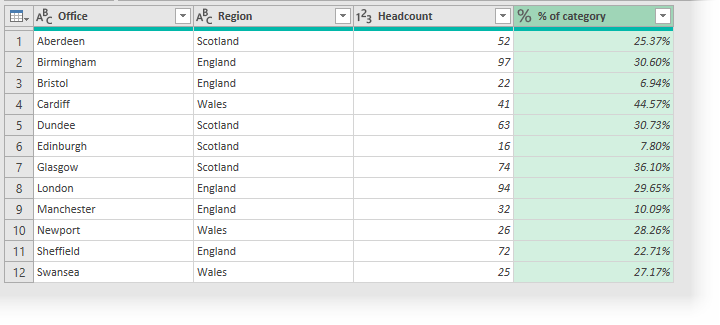The process was similar to calculating the percent of a total, but the formula you pasted is much more complex. In the sections below, we’ll dig a bit deep to understand how it works.  This will enable you to create this transformation for yourself.

## How % of total works

Power Query, itself does not have a total row.  This isn’t a problem, as the tool is intended for data manipulation, rather than presentation.  But it does mean there is not a total row to divide by.

List.Sum is similar to Excel’s SUM function.  The following M code uses that formula to calculate the total value for the Headcount column, using the #”Changed Type” step as its source.

`List.Sum(#"Changed Type"[Headcount])`

Having calculated the total, we just need to divide the number by the result of the List.Sum function result.

`=[Headcount] / List.Sum(#"Changed Type"[Headcount])`

Pretty easy, right?

100 Excel VBA Macros

Do you know the fastest way to learn foreign languages?  It is to read, write, speak, and think in that language as often as possible.  Apart from speaking, programming languages are no different.  The more you immerse yourself in that language, the faster you will pick it up.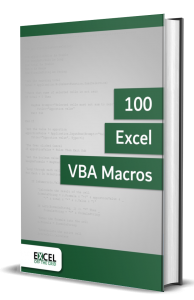Therefore, what most people like you need is lots of examples that you can practice.  That is why the 100 Excel VBA Macros eBook exists.  It’s the book for all Excel users who want to learn how to read and write Excel macros, save time, and stand out from their peers.  The book contains:

• 100 example codes to practice reading and writing macros that will embed the language into your thinking.
• An introduction to macros in Excel to ensure you can implement the VBA code in the book even if you have no prior knowledge.
• Consistent code layout between examples to enable you to understand the structure and easily customize the code to meet your needs.
• Downloadable workbook containing all the source code, so the examples can be added to your project to give you the benefit of VBA straight away.

## How % of category works

Calculating the % of a category is a little tricky.  We have seen this scenario previously when we looked at custom functions.  While a custom function is an option, we can also achieve the result (and possibly faster and easier) by nesting transformations into a single formula.

We will step through the transformations one-by-one so that we can understand how the technique works.

#### Step 1

Begin with the source table loaded into Power Query.#### Step 2

Click Transform -> Group By from the Power Query ribbon.The Group By dialog box will open.  Enter the following information:

• View: Basic
• Column: Region (i.e., the column which contains the category column)
• New column name: Any name you want.  Given our data set, Total Region seems sensible
• Operation: Sum – the calculation we want to perform
• Column: Headcount – the column containing the numbers we want to perform the operation onClick OK to close the dialog box.

Look at the formula bar at the top of the Preview Window (click View -> Formula Bar if it is not visible).  The M code for this step looks like this:

```= Table.Group(#"Changed Type", {"Region"},
{{"Total Region", each List.Sum([Headcount]), type number}})```

The Table.Group function is the M code which executes the Group By transformation.  Learn more about the Table.Group function here: https://docs.microsoft.com/en-gb/powerquery-m/table-group

#### Step 3

Filter the category column to include a single value.  I have selected Wales.  Then click OK.The M code for this step is:

`= Table.SelectRows(#"Grouped Rows", each ([Region] = "Wales"))`

The Table.SelectRows function is the M code that executes a column filter.  Learn more about the Table.SelectRows function here: https://docs.microsoft.com/en-gb/powerquery-m/table-selectrows

#### Step 4

Now let’s combine the two transformations from Step 2 and Step 3 into a single formula.

#”Grouped Rows” is the reference to the step above.  So, we just need to make two simple changes:

• Add the text before the step name to the beginning of the step above
• Add the text after the step name to the end of the step above

The M code now looks like this:

```= Table.SelectRows(
Table.Group(#"Changed Type", {"Region"},
{{"Total Region", each List.Sum([Headcount]), type number}})
, each ([Region] = "Wales"))```
• Red is the transformation from Step 2
• Blue is the code added from Step 3

As the last step has now been added into the step above, we can delete the last step.

The preview window now only has one line of data.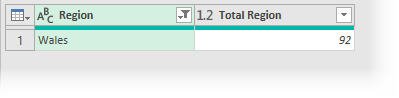#### Step 5

Right-click on the value within the Total Region column and select Drill Down from the menu.The M code for this step looks like this:

`= #"Grouped Rows"{[Region="Wales"]}[Total Region]`

#### Step 6

Now let’s add the code from step 5 into Step 4.

The M code now looks like this:

```= Table.SelectRows(
Table.Group(#"Changed Type", {"Region"},
{{"Total Region", each List.Sum([Headcount]), type number}})
, each ([Region] = "Wales")){[Region="Wales"]}[Total Region]```
• Red is the transformation from Step 2
• Blue is the added code from Step 3
• Magenta is the added code from Step 5

As the drill down has now been incorporated into the step above, we can delete the last step from the applied steps window.

#### Step 6

We now have all the transformations required, so it’s time to turn the formula into its own column.

1. Copy all the text for the combined formula from the Formula Bar.
2. Delete the step containing the formula from the Applied Steps list.
3. Click Add Column -> Custom Column
4. Paste the copied text into the Custom Column dialog box.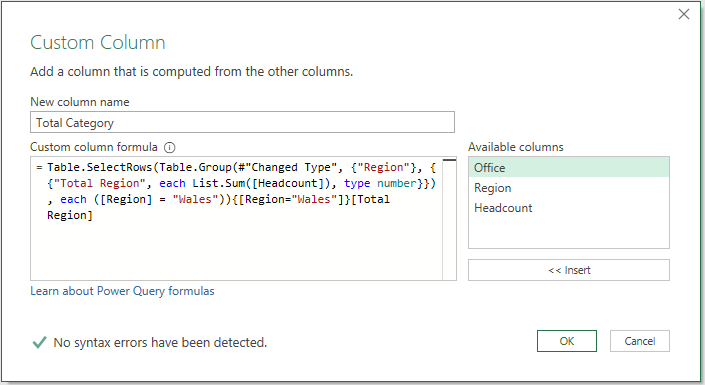5. Replace each instance of “Wales” with [Region].  The code now looks like this (changes highlighted).  Obviously, you’ll adapt this to your scenario.
```=Table.SelectRows(
Table.Group(#"Changed Type", {"Region"},
{{"Total Region", each List.Sum([Headcount]), type number}})
, each ([Region] = [Region])){[Region=[Region]]}[Total Region]```
6. Finally, add the column to be divided to the start of the formula
```=[Headcount] / Table.SelectRows(
Table.Group(#"Changed Type", {"Region"},
{{"Total Region", each List.Sum([Headcount]), type number}})
, each ([Region] = [Region])){[Region=[Region]]}[Total Region]```

That’s it; we now have a % of the region for every row.## Conclusion

In this post, we focused on how to calculate the % of a total and % of a category.  Through this, we learned about the List.Sum function (which is similar to Excel’s SUM function) and also how to combine queries into a single transformation step. This is a great technique to achieve more advanced transformations.Get our FREE VBA eBook of the 30 most useful Excel VBA macros.
Automate Excel so that you can save time and stop doing the jobs a trained monkey could do.

Email Address * First Name *

Don’t forget:

I’m guessing the examples in this post didn’t exactly meet your situation.  We all use Excel differently, so it’s impossible to write a post that will meet everybody’s needs.  By taking the time to understand the techniques and principles in this post (and elsewhere on this site) you should be able to adapt it to your needs.

But, if you’re still struggling you should:

1. Read other blogs, or watch YouTube videos on the same topic.  You will benefit much more by discovering your own solutions.
2. Ask the ‘Excel Ninja’ in your office.  It’s amazing what things other people know.
3. Ask a question in a forum like Mr Excel, or the Microsoft Answers Community.  Remember, the people on these forums are generally giving their time for free.  So take care to craft your question, make sure it’s clear and concise.  List all the things you’ve tried, and provide screenshots, code segments and example workbooks.
4. Use Excel Rescue, who are my consultancy partner.   They help by providing solutions to smaller Excel problems.

What next?
Don’t go yet, there is plenty more to learn on Excel Off The Grid.  Check out the latest posts:

## 11 thoughts on “Power Query – Percent of total or category”

1.Andrew Vachris says:

Hi – the How % of category seems a bit cumbersome – wouldn’t we be better served by breaking this up into steps? For example do a group by sum, and then bring that value back via a merge?

I’m not sure anyone would be able to do this otherwise by free handing this.

•Excel Off The Grid says:

There are many approaches to do this.

Since the M code is recorded as you undertake each step, then it’s not too difficult to work out. Once you understand the technique then you can apply it in many scenarios.

2.Hugo says:

This post is simply fantastic. Thank you so much.

•Excel Off The Grid says:

Thanks Hugo 🙂

3.korea says:

The problem has been solved. Thank you.

4.Jim says:

If i want to group by two categories, where in the code do i put that?

5.Jim says:

Trying to use your method to build my string so this part works for Step 6 no.5

= Table.SelectRows(Table.Group(#”Added Custom3″, {“UNIQUE_KEY”, “AS_OF_MONTH”}, {{“TOTAL_CURRENT_BALANCE”, each List.Sum([CURRENT_BALANCE]), type nullable number}}), each ([UNIQUE_KEY] = “5841” and [AS_OF_MONTH] = #date(2018, 6, 30))){[UNIQUE_KEY=”5841″, AS_OF_MONTH=#date(2018, 6, 30)]}[TOTAL_CURRENT_BALANCE]

But when I replace my values with the fields i get the error: Expression.Error: There is an unknown identifier. Did you use the [field] shorthand for a _[field] outside of an ‘each’ expression?

= Table.SelectRows(Table.Group(#”Added Custom3″, {“UNIQUE_KEY”, “AS_OF_MONTH”}, {{“TOTAL_CURRENT_BALANCE”, each List.Sum([CURRENT_BALANCE]), type nullable number}}), each ([UNIQUE_KEY] = [UNIQUE_KEY] and [AS_OF_MONTH] = [AS_OF_MONTH])){[UNIQUE_KEY=[UNIQUE_KEY], AS_OF_MONTH=[AS_OF_MONTH]]}[TOTAL_CURRENT_BALANCE]

6.Jim says:

Alright figure it out, I thought I was just replacing my previous step with the code from step 6 part 5, realized it didn’t include an actual add column statement. This work awesome, thanks for the tutorial.

Table.Group(#”Added Custom3″, {“UNIQUE_KEY”, “AS_OF_MONTH”}, {{“TOTAL_BALANCE”, each List.Sum([CURRENT_BALANCE]), type nullable number}})
, each ([UNIQUE_KEY] = [UNIQUE_KEY] and [AS_OF_MONTH] = [AS_OF_MONTH])){[UNIQUE_KEY=[UNIQUE_KEY],AS_OF_MONTH=[AS_OF_MONTH]]}[TOTAL_BALANCE])

7.Matt says:

Hi,

How could I do the same thing but on a grouping of TWO columns. For example, you had an extra column MONTH and wanted to percentage headcount of total REGION-MONTH combination.

Thank you

8.Miyan says:

Would it be possible to more than one level of categorization. Lets say you wanted to break it down further down to state or county.

Region>State>County

Table.Group(#”Added Conditional Column2″, {“Region”, “State”, “County”}

How would you alter the remaining part of the Formula?

•Excel Off The Grid says:

Yes, it is possible. I would recommend re-loading the data, go through the grouping with multiple steps using the interface. Then look at the M code structure, which should give you the structure to answer your question.